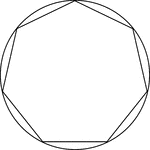### Regular Heptagon/Septagon Inscribed In A Circle

Illustration of a regular heptagon/septagon inscribed in a circle. This can also be described as a circle…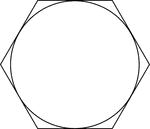### Regular Hexagon Circumscribed About A Circle

Illustration of a regular hexagon circumscribed about a circle. This can also be described as a circle…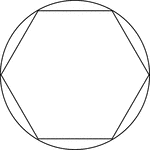### Regular Hexagon Inscribed In A Circle

Illustration of a regular hexagon inscribed in a circle. This can also be described as a circle circumscribed…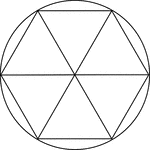### Regular Hexagon Inscribed In A Circle

Illustration of a regular hexagon inscribed in a circle. This can also be described as a circle circumscribed…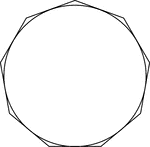### Regular Nonagon Circumscribed About A Circle

Illustration of a regular nonagon circumscribed about a circle. This can also be described as a circle…### Regular Nonagon Inscribed In A Circle

Illustration of a regular nonagon inscribed in a circle. This can also be described as a circle circumscribed…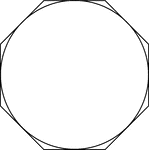### Regular Octagon Circumscribed About A Circle

Illustration of a regular octagon circumscribed about a circle. This can also be described as a circle…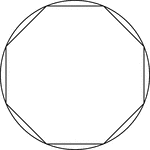### Regular Octagon Inscribed In A Circle

Illustration of a regular octagon inscribed in a circle. This can also be described as a circle circumscribed…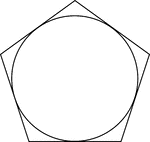### Regular Pentagon Circumscribed About A Circle

Illustration of a regular pentagon circumscribed about a circle. This can also be described as a circle…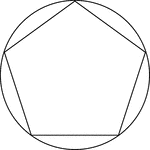### Regular Pentagon Inscribed In A Circle

Illustration of a regular pentagon inscribed in a circle. This can also be described as a circle circumscribed…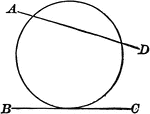### Circle with Secant and Point of Tangency

Illustrations of a circle with secant AD and line BC tangent to it.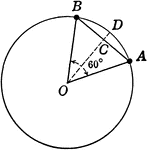### Circle With Sector and Segment labeled

Illustration of circle with sector and segment used to find area.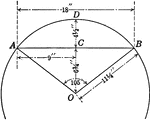### Circle With Sector and Segment labeled

Illustration of partial circle with sector and segment used to find area.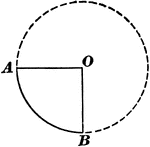### Sector of Circle

Illustration of a circle with a sector. "A sector of a circle is the space included between an arc and…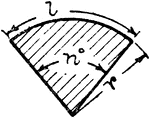### Sector Of Circle

Illustration of a sector of a circle. A sector is the space between an arc and two radii drawn to the…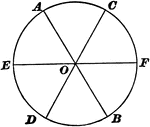### Sectors of Circle

Illustration of a circle with 6 equal sectors.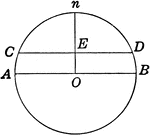### Circle With Diameter and Segment labeled

Illustration of circle with diameter and segment used to find area.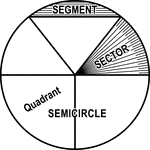### Circle with Segment, Semicircle, Sector, Quadrant, and Central Angle

Illustrations of a circle with segment, semicircle, sector, quadrant, and central angles drawn.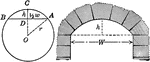### Segments of Circle and Arch

Illustration of circle with segments labeled and arch.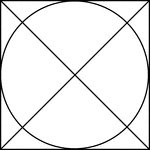### Square Circumscribed About A Circle

Illustration of a square, with diagonals drawn, circumscribed about a circle. This can also be described…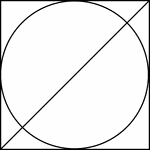### Square Circumscribed About A Circle

Illustration of a square, with 1 diagonals drawn, circumscribed about a circle. This can also be described…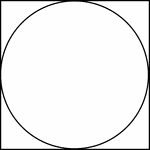### Square Circumscribed About A Circle

Illustration of a square circumscribed about a circle. This can also be described as a circle inscribed…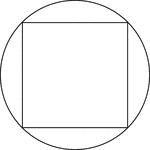### Square Inscribed In A Circle

Illustration of a square inscribed in a circle. This can also be described as a circle circumscribed…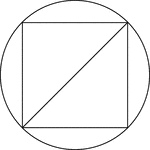### Square Inscribed In A Circle

Illustration of a square inscribed in a circle. This can also be described as a circle circumscribed…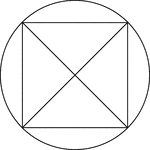### Square Inscribed In A Circle

Illustration of a square, with diagonals drawn, inscribed in a circle. This can also be described as…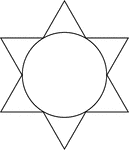### Star Circumscribed About A Circle

Illustration of a 6-point star (convex dodecagon) circumscribed about a circle. This can also be described…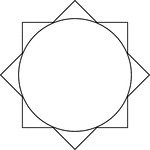### Star Circumscribed About A Circle

Illustration of an 8-point star (convex polygon) circumscribed about a circle. This can also be described…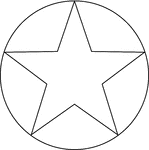### Star Inscribed In A Circle

Illustration of a 5-point star inscribed in a circle. This can also be described as a circle circumscribed…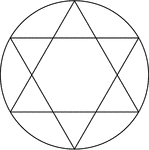### Star Inscribed In A Circle

Illustration of a 6-point star created by two equilateral triangles (often described as the Star of…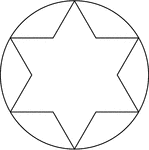### Star Inscribed In A Circle

Illustration of a 6-point star (convex dodecagon) inscribed in a circle. This can also be described…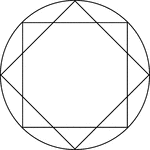### Star Inscribed In A Circle

Illustration of an 8-point star, created by two squares at 45° rotations, inscribed in a circle.…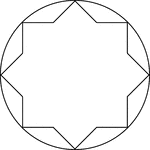### Star Inscribed In A Circle

Illustration of an 8-point star, or convex polygon, inscribed in a circle. This can also be described…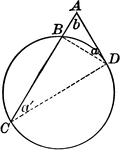### Circle With Tangent and Secant

Circle with secant and tangent drawn.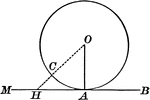### Circle With Tangent Line Drawn

Illustration of a circle with a tangent drawn - a straight line perpendicular to a radius at its extremity.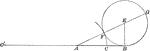### Circle and Triangle

Circle with triangle to show how to divide a line in extreme and mean ratio.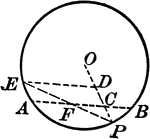### Circle and Triangle

Circle with triangle and chords.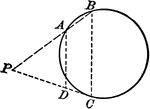### Circle and Triangle

Circle with triangle and chords.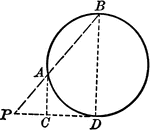### Circle and Triangle

Circle with triangle and chords.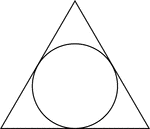### Triangle Circumscribed About A Circle

Illustration of an equilateral triangle circumscribed about a circle. This can also be described as…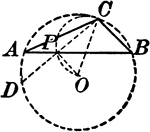### Circle and Inscribed Triangle

Circle with triangle inscribed.### Triangle Inscribed in Circle

Illustration of triangle inscribed in circle. Or, circle circumscribed about triangle.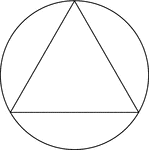### Triangle Inscribed In A Circle

Illustration of an equilateral triangle inscribed in a circle. This can also be described as a circle…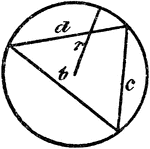### Triangle Inscribed In A Circle

An illustration showing a triangle with sides b, c, and d inscribed in a circle with radius r.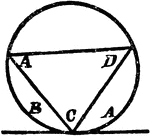### Triangle Inscribed In A Circle

An illustration showing a triangle with angles A, C, and D inscribed in a circle which is tangent to…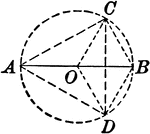### Circle With Inscribed Triangle and Radii

Illustration showing a circle with an inscribed triangle and radii.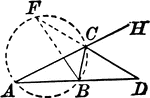### Circle and Triangles

Circle with two triangles.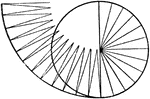### Triangles Making Up A Circle

Illustration showing that a circle may be considered as made up of triangles whose bases form the circumference.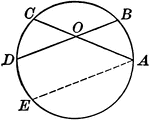### Circle With Two Intersecting Chords

Illustration of a circle with two intersecting chords within the circumference. The angle formed is…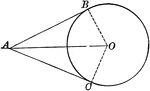### Circle With Two Tangents Drawn From an External Point

Illustration of a circle which illustrates that the tangents to a circle drawn from an external point…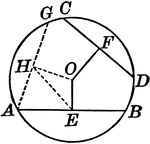### Circle With Unequal Chords

Illustration of a circle with unequal chords, which are unequally distant from the center.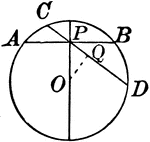### Circle With Various Chords

Illustration showing a circle with various chords drawn.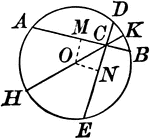### Circle With Various Chords

Illustration showing a circle with various chords drawn.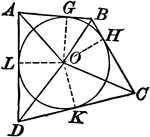### Circle With Various Chords And Tangents

Illustration showing a circle with various chords and tangents drawn.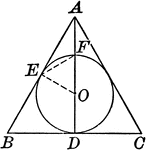### Circle With Various Chords And Tangents

Illustration showing a circle with various chords and tangents drawn.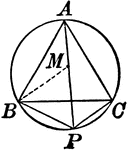### Circle With Inscribed Equilateral Triangle

Illustration showing an equilateral triangle inscribed in a circle.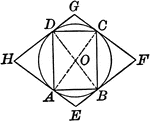### Circle With Inscribed and Circumscribed Quadrilaterals

Illustration showing a circle with an inscribed and circumscribed quadrilateral.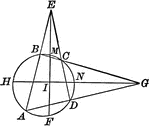### Circle With Inscribed Quadrilateral and Triangles Formed

Illustration showing a circle with an inscribed quadrilateral and triangles formed by extended chords.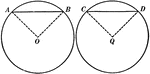### Equal Chords in Equal Circles Theorem

Illustration used to show that "In equal circles, or in the same circle, if two chords are equal, they…